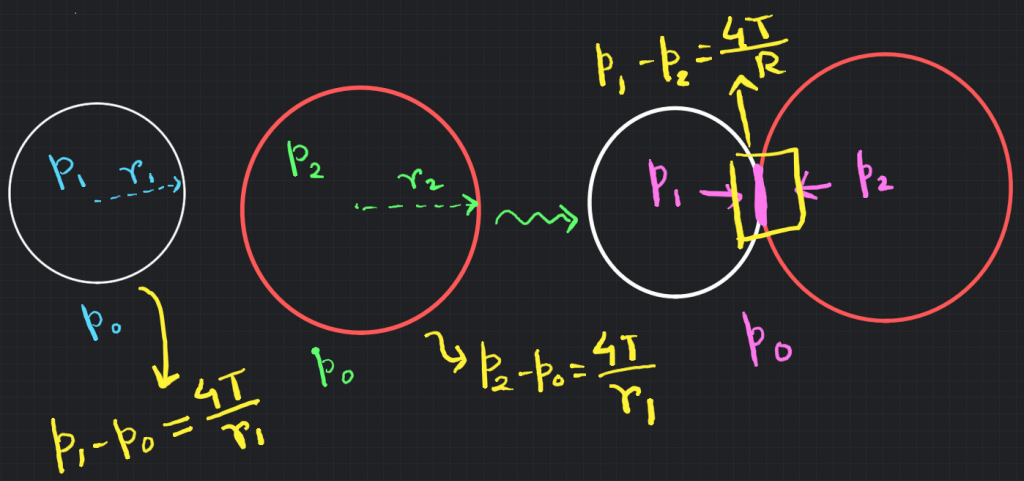How Cheenta works to ensure student success?
Explore the Back-Story

# NSEP 2015 Problem 6 | Surface Tension and PressureTry out this problem on the Surface Tension and Pressure from National Standard Examination in Physics 2015-2016.

## NSEP 2015-16 ~ Problem 6

Two air bubble with radius $r_1$ and $r_2$ $(r_2>r_1)$ formed of the same liquid stick to each other to form a common interface. Therefore, the radius of curvature of the common surface is

(a) $\sqrt{r_1 r_2}$ (b) Infinity (c) $\frac{r_2}{r_1}\sqrt{{r_2}^2-{r_2}^2}$ (d) $\frac{r_1 r_2}{r_2 - r_1}$

### Key Concepts

Basic surface Tension

Relation between surface Tension and Radius of curvature

## Suggested Book | Source | Answer

Concept of Physics H.C. Verma

University Physics by H. D. Young and R.A. Freedman

Fundamental of Physics D. Halliday, J. Walker and R. Resnick

National Standard Examination in Physics(NSEP) 2015-2016

Option-(d) $\frac{r_1 r_2}{r_2 - r_1}$

## Try with Hints

For a bubble of radius $r$ with double surface and whose inside pressure is $p_{in}$ and outside pressure is $p_{out}$ and also the surface tension is $T$, the relation between pressure and radius is,

$$p_{in} - p_{out} = \Delta p = \frac{4T}{r}$$

When 2 bubble merge on their common interface the pressure difference is Just the difference between the pressure(inside) of both two bubbles. Also, the surface tension remains same.

We know $p_1 - p_0 = \frac{4T}{r_1}$ and $p_2 - p_0 = \frac{4T}{r_2}$, Then,

$$p_1 - p_2 = \frac{4T}{R}$$

here $R$ is the radius of curvature of the interface.

Hence,

$p_{1}-p_{2}$=$\left(p_{1}-p_{0}\right)-\left(p_{2}-p_{0}\right)$=$4 T\left(\frac{1}{r_{1}}-\frac{1}{r_{2}}\right)$

This gives us,

$$\frac{1}{R} = \frac{1}{r_1}-\frac{1}{r_2} \to R= \frac{r_1 r_2}{r_2 - r_1}$$

## Subscribe to Cheenta at Youtube

Try out this problem on the Surface Tension and Pressure from National Standard Examination in Physics 2015-2016.

## NSEP 2015-16 ~ Problem 6

Two air bubble with radius $r_1$ and $r_2$ $(r_2>r_1)$ formed of the same liquid stick to each other to form a common interface. Therefore, the radius of curvature of the common surface is

(a) $\sqrt{r_1 r_2}$ (b) Infinity (c) $\frac{r_2}{r_1}\sqrt{{r_2}^2-{r_2}^2}$ (d) $\frac{r_1 r_2}{r_2 - r_1}$

### Key Concepts

Basic surface Tension

Relation between surface Tension and Radius of curvature

## Suggested Book | Source | Answer

Concept of Physics H.C. Verma

University Physics by H. D. Young and R.A. Freedman

Fundamental of Physics D. Halliday, J. Walker and R. Resnick

National Standard Examination in Physics(NSEP) 2015-2016

Option-(d) $\frac{r_1 r_2}{r_2 - r_1}$

## Try with Hints

For a bubble of radius $r$ with double surface and whose inside pressure is $p_{in}$ and outside pressure is $p_{out}$ and also the surface tension is $T$, the relation between pressure and radius is,

$$p_{in} - p_{out} = \Delta p = \frac{4T}{r}$$

When 2 bubble merge on their common interface the pressure difference is Just the difference between the pressure(inside) of both two bubbles. Also, the surface tension remains same.

We know $p_1 - p_0 = \frac{4T}{r_1}$ and $p_2 - p_0 = \frac{4T}{r_2}$, Then,

$$p_1 - p_2 = \frac{4T}{R}$$

here $R$ is the radius of curvature of the interface.

Hence,

$p_{1}-p_{2}$=$\left(p_{1}-p_{0}\right)-\left(p_{2}-p_{0}\right)$=$4 T\left(\frac{1}{r_{1}}-\frac{1}{r_{2}}\right)$

This gives us,

$$\frac{1}{R} = \frac{1}{r_1}-\frac{1}{r_2} \to R= \frac{r_1 r_2}{r_2 - r_1}$$

## Subscribe to Cheenta at Youtube

This site uses Akismet to reduce spam. Learn how your comment data is processed.

### Knowledge Partner